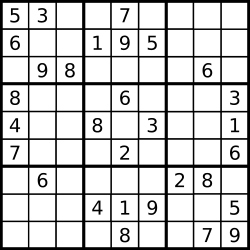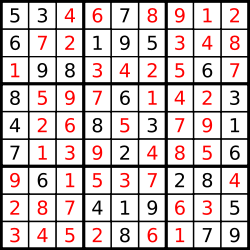# 算法-数独

本文为「原创」内容，如需转载请注明出处！

### 背景

leetcode 链接

1. 给定一个只含有 1-9 数字的宫格2. 要求每一行、每一列、每一个 3x3 的小格子里面的数字不重复### 算法

1. 找到所有未被填充数字的宫格，用 bit 记录每行，每列，每个 3x3 格子的状态
2. 通过 DFS 来给未被填充的格子逐个试可用的数字，直到所有格子填充完毕
tip:
某位为 1 表示已经含有该位的数字
row = 11001      表示第一行已经含有数字 1、4、5
col = 11001      表示第一列已经含有数字1、4、5
block = 11001 表示第一个 3x3 格子已经含有数字1、4、5


### 实现

    public void solveSudoku(char[][] board) {
int[] rows = new int;
int[] cols = new int;
int[][] block = new int;
List<Integer> miss = new ArrayList<>();
for (int i = 0; i < 9; ++i) {
for (int j = 0; j < 9; ++j) {
if (board[i][j] != '.') {
int num = board[i][j] - '1';
rows[i] |= (1 << num);
cols[j] |= (1 << num);
block[i / 3][j / 3] |= (1 << num);
} else {
}
}
}
dfs(board, rows, cols, block, miss, 0);
}

private boolean dfs(char[][] board, int[] rows, int[] cols,
int[][] block, List<Integer> miss, int start) {
if (start == miss.size()) {
return true;
}
int index = miss.get(start);
int y = index / 9;
int x = index % 9;
int full = 0b111111111;
// 找到所有为 0 的位，并将其置位 1
int candidate = full ^ (rows[y] | cols[x] | block[y / 3][x / 3]);
while (candidate > 0) {
// 取出最后一个不为 0 开始的数字
// 比如 candidate = 10100 那么 bit 则为 100
int bit = candidate & (-candidate);
rows[y] |= bit;
cols[x] |= bit;
block[y / 3][x / 3] |= bit;
if (dfs(board, rows, cols, block, miss, start + 1)) {
// 找到候选值表示的数字，具体去请看上面的 tip
// 比如 bit = 100  那么 bit 表示的数字是 3
int num = 1;
while(bit>>1 != 0){
bit>>=1;
num+=1;
}
board[y][x] = (char)(num + '0');
return true;
}
// 进入到了这里，说明前面的候选值不满足
// 删除之前的候选值，进入下一个候选值
candidate -= bit;
rows[y] -= bit;
cols[x] -= bit;
block[y / 3][x / 3] -= bit;
}
return false;
}